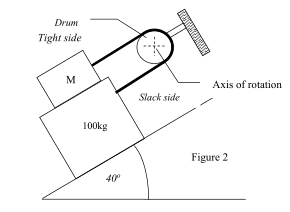# Capstan law forces

• I
Hello!

Capstan law is T2=T1e^μθ.

I have problem identifying T1 and T2 in various situations:

1) when the capstan is not rotating
2) when the capstan is rotating

how can I identify which side is T1 and which T2?

thanks!

billy_joule
Naming the forces descriptively rather than numbers may help:

It doesn't matter whether the capstan is rotating or not. Identifying which is Tload and which is Teffort should be obvious in most cases.

I still don't understand what is load and what is effort. How can I determine that in a situation like the below?Is it always the heavier load the Tload and the lighter Teffort? What if it is not given which is heavier and which lighter? Or if a heavier object is at an incline which makes the net force smaller?

billy_joule
I still don't understand what is load and what is effort.
https://en.wikipedia.org/wiki/Capstan_equation

How can I determine that in a situation like the below?

Well your picture identifies which is the tight and slack which I would expect to correspond to load and effort. Tight being high tension = load, slack being low tension = effort. In other words M > 100kg.
They could just have easily reversed the tight and slack side labelling in which case M < 100kg.
If the tight and slack side were not labelled at all you could find the max M value (>100kg), which corresponds to M being the load, and the minimum M value (<100kg) which corresponds to M being the effort.
The weights will be stationary if M is anywhere between these two limits.

S you are saying that the biggest force is always the Tload and the smaller force is Teffort? If yes, nice, that's what I wanted: a rule to decide which is which.

billy_joule
S you are saying that the biggest force is always the Tload and the smaller force is Teffort? If yes, nice, that's what I wanted: a rule to decide which is which.
Yes, that's right.

sophiecentaur
Gold Member
S you are saying that the biggest force is always the Tload and the smaller force is Teffort? If yes, nice, that's what I wanted: a rule to decide which is which.
Work put in times efficiency = Work got out
or
Feffort times distance moved times Efficiency = Fload times distance moved
Efficiency is always less than one.

Work put in times efficiency = Work got out
or
Feffort times distance moved times Efficiency = Fload times distance moved
Efficiency is always less than one.

thanks but irrelevant with the topic

sophiecentaur
Gold Member
thanks but irrelevant with the topic
I would say that is very relevant because it determines which way the energy is flowing. Isn't that relevant to the thread?

Who asked about energy? There is no energy involved as there is no movement.

sophiecentaur
Gold Member
Who asked about energy? There is no energy involved as there is no movement.
The terms Load and Effort refer to a machine. That is defined as an arrangement to do Work - aka transfer Energy. If there is no 'defined' direction for the direction of transfer then you can't say which is the load and which is the effort. That was the message in the OP. When the capstan is rotating there is energy involved.
When there is no movement, you can use the idea of Virtual Work, where an infinitesimal movement is allowed. I remember doing that at A level - but not since.

I am not sure what you did at A levels
Here it is supposed that the effort force is less than the load force, so the efficiency of the capstan is above 1

sophiecentaur